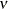The COUNTREG Procedure

PRIOR Statement

(Experimental)

• PRIOR _REGRESSORS |parameter-list ~ distribution ;

The PRIOR statement specifies the prior distribution of the model parameters. You must specify a single parameter or a list of parameters, a tilde (~), and then a distribution with its parameters. Multiple PRIOR statements are allowed.

You can specify the following distributions:

BETA(SHAPE1=a, SHAPE2=b, MIN=m, MAX=M)

specifies a beta distribution that has the parameters SHAPE1 and SHAPE2 and is defined between MIN and MAX.

GAMMA(SHAPE=a, SCALE=b)

specifies a gamma distribution that has the parameters SHAPE and SCALE.

IGAMMA(SHAPE=a, SCALE=b)

specifies an inverse gamma distribution that has the parameters SHAPE and SCALE.

NORMAL(MEAN=, VAR=)

specifies a normal distribution that has the parameters MEAN and VAR.

T(LOCATION=, DF=)

specifies a noncentral t distribution that has DF degrees of freedom and a location parameter equal to LOCATION.

UNIFORM(MIN=m, MAX=M)

specifies a uniform distribution that is defined between MIN and MAX.